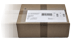# Casio FX-5800P

CHF 75.00When you order this item your purchase will be despatched free or charge
Availability
Available from stock  Delivery within 1-3 working days!
Manufacturer
Casio
Guarantee
36 Months
Dimensions
82 x 163 x 18 mm
Weight
150 g
Directions for Use
German, English, Italian, Spanish

#### Items delivered

• Casio FX-5800P
• Protective cover (swings back a full 360°)
• Battery (1 x AAA)
The Casio FX-5800P makes programming easy: the FX-5800P helps you create complex programmes and calculate complex numbers
• Natural dot matrix display (4 lines with 16 characters)
• 24 levels of parentheses
• S.V.P.A.M. (Super Visual Perfect Algebraic Method)
• 26 memories
• 40 physical constants
• 128 built-in formulae
• Complex number calculation
• Trigonometry functions
• Algebraic entry logic
• Automatic fraction calculation
• Function calculations
• Calculations using irrational values (?)
• CALC function
• Resistance calculations
• Error correction function
• Base n calculations (BIN/OCT/DEC/HEX)
• Statistical calculations (statistics based on 1 and 2 lists, Regression calculations, Normal distribution)
• Equation solver for equation systems with 2 and 3 unknowns, polynomic equations (2nd & 3rd degree)
• Calculates matrices (max. 10 x 10)
• Numerical calculations for value tables
• Recursive calculations for value tables
• BASIC-like programme functions (IF etc.)
• Program memory 28kB
• Back up function (Flash Memory)
• Communication via 3 pin cable (calculator to calculator, requires optional SB-62 cable)

Casio approval regulations Switzerland for bulk school orders (PDF)
Accessories

^ top